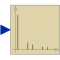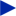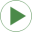# RealFFT

Sampling and FFT of input u# Information

This information is part of the Modelica Standard Library maintained by the Modelica Association.

This block samples the input signal, calculates the Fast Fourier Transform by function Math.realFFT, and (when simulation terminates) writes the output to result file resultFileName by function Math.realFFTwriteToFile.

The number of sampling points as well as the samplePeriod is calculated from desired maximum frequency f_max and frequency resolution f_res.

#### Note

The user has to take care that enough points can be sampled before the simulation ends: startTime + (ns - 1)*samplePeriod <= stopTime.

The result file is written as mat, first column = frequency, second column = amplitudes, third column = phases. The frequency points are separated by rows with amplitude and phase = 0, so one can plot the result directly as frequency lines.

# Parameters (7)

samplePeriod Value: 1 / (2 * f_res * div(ns, 2)) Type: Time (s) Description: Sample period of component Value: 0 Type: Time (s) Description: First sample time instant Value: Type: Frequency (Hz) Description: Maximum frequency of interest Value: Type: Frequency (Hz) Description: Frequency resolution Value: Modelica.Math.FastFourierTransform.realFFTsamplePoints(f_max, f_res, f_max_factor = 5) Type: Integer Description: Number of samples Value: max(1, min(integer(ceil(f_max / f_res)) + 1, div(ns, 2))) Type: Integer Description: Number of frequency points Value: "realFFT.mat" Type: String Description: Result file: f, abs, arg

# Outputs (3)

sampleTrigger Type: Boolean Description: True, if sample time instant Type: Boolean Description: Rising edge signals first sample instant Type: Integer Description: Information flag from FFT computation

# Connectors (1)

 uType: RealInput

# Used in Examples (2)Rectifier6pulseFFT Modelica.Blocks.Examples Example of FFT blockRectifier12pulseFFT Modelica.Blocks.Examples Example of FFT block